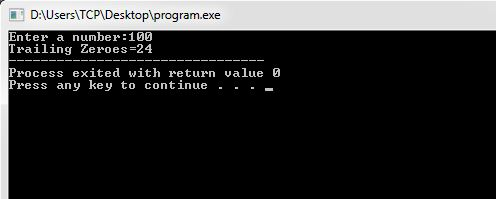# Count Trailing Zeros in Factorial of Number

Here you will learn about how to count trailing zeros in factorial of number.

One simple approach to count trailing zeros is first find factorial of number and then count the zeros in the factorial one by one. It is fine for smaller number like 10.

10! = 3628800

So, trailing zeros = 2

But what about big numbers like 100. The factorial of 100 has 24 zeros in the end and almost 160 digits. Its really hard to store that big number and then count the zeros one by one.

There is a simple and very fast method to do this. We can count the zeros by counting the 5s in prime factor of n factorial.

It is very frequently asked question in competitive programming. Let us see how this method can be implemented in C.

## C Program to Count Trailing Zeros in Factorial of Number

OutputIf you have any doubts regarding above tutorial then comment below.

### 7 thoughts on “Count Trailing Zeros in Factorial of Number”

1.Thanks for sharing!
Is there any reason why we divide by factors of 5 in each iteration?

1.Its just an algorithm, we have to follow it.

1.@Admin.- With all due respect , there is a reason for every algorithmic design that works 🙂 @Aufuray – Do keep in mind that 5 and 2 are the parents of 10 (i.e they literally give birth to 10 when multiplied together )

So, what we are basically doing in this algo , is that we are counting the number of fives that are being multiplied .. ( Wondering why we will not count the 2’s ? Because 2 ‘s will always be more than the number of 5’s needed to make a 10 .. but as 5 is the limiting determinant to make a 10 , therefore we count the number of 5’s)
More mathematical explanation –>http://mathworld.wolfram.com/Factorial.html

2.I’m impressed, I must say. Rarely do I come across a blog that’s both educative and interesting,
and without a doubt, you’ve hit the nail on the head.

The issue is something which too few people are speaking intelligently about.
I am very happy that I stumbled across this during my hunt for something relating to this.

3.Wow, Nice!

4.can u xplain about the logic

1.With all due respect , there is a reason for every algorithmic design that works 🙂 @ram – Do keep in mind that 5 and 2 are the parents of 10 (i.e they literally give birth to 10 when multiplied together )

So, what we are basically doing in this algo , is that we are counting the number of fives that are being multiplied .. ( Wondering why we will not count the 2’s ? Because 2 ‘s will always be more than the number of 5’s needed to make a 10 .. but as 5 is the limiting determinant to make a 10 , therefore we count the number of 5’s)
More mathematical explanation –>http://mathworld.wolfram.com/Factorial.html